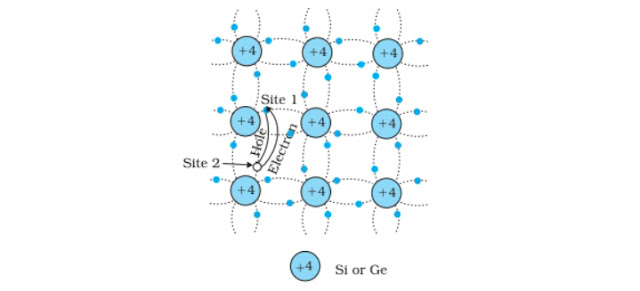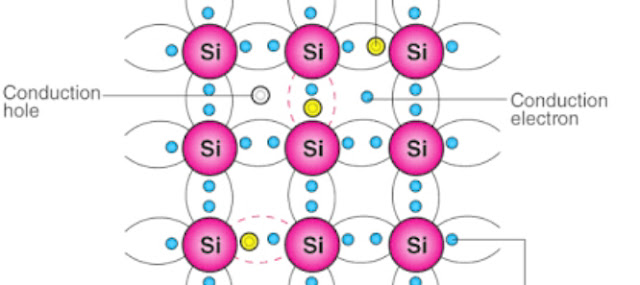# semiconductor- types and behavior of semiconductors

Views

### Semiconductor

Semiconductor has been playing a major role in electronics. They have made it possible for engineers and scientists to develop light and small type of devices. That's why in today's article I would like to share with you an interesting topic named "Semiconductor" . The article will also discuss  about the types of Semiconductor

#### What are semiconductors?

Metals having conductivity in between conductors and insulators , we call them as Semiconductors

Talking about the energy band diagram of Semiconductors , the valence band is completely filled and the conduction band is completely empty. The energy gap is small nearly equal to one electron volt.  I would like to clear your confusion with a simple example; The energy gap of germanium is 0.78 ev and the energy gap of silicon is 1.21 ev.

#### I)  Behavior at absolute zero temperature:-

when electric field is applied, then the valence electrons will not reach from valence band to conduction band and hence no current flows through the semi conductor. In other words we can say that ,it behaves like insulator at 0k.

#### II) Behavior at room temperature :-

When electric field is applied, the valence electrons will reach from valence band to conduction band and hence there is flow of current through the Semiconductor. In other words, They behave as conductor at room temperature.

#### Types of semi-conductors:

There are two types of semi-conductors discovered so far, one is Intrinsic semiconductor and the other one Extrinsic.let's discuss these two types separately:

#### Intrinsic Semiconductor :

A pure semiconductor which is free from impurities is called an intrinsic semiconductor.Intrinsic semiconductor

For example;  Germanium and silicon are the important examples of intrinsic Semiconductors

Let's see the electronic configurations of silicon and germanium ;

Silicon (14) ; 1S², 2S² , 2P⁶,3S² , 3P²

Germanium (32) ; 1S², 2S² , 2P⁶,3S² , 3P⁶,3d¹⁰, 4S², 4P².

Thus it is clear that there are four valence electrons in silicon or germanium.

The crystal structure of silicon (14) is shown in the figure.

Here the four valence electrons of silicon atom, form four covalent bonds . This happens when they share the four electrons belonging to four neighbouring silicon atoms. After the formation of such covalent bonds, each silicon atom in the crystal structure behaves like its outermost orbit is complete with 8 electrons.

#### Behavior at zero Kelvin temperature :-

All the electrons are rigidly bound to their nuclei, when electric field is applied. We don't have free electrons to move from valence band to conduction band. Therefore, it is clear that a Semiconductor behaves as an insulator at very low temperature.

#### Behavior of  Semiconductors at room temperature :-

When electric field is applied, some of the electrons are detached from covalent bonds and they have enough energy to to go from valence band to conduction band and hence large amount of current flows through Semiconductor at room temperature.

we also note that,  when we increase the temperature of a semi-conductors, then the number of valence electrons going from valence band to conduction band also increases and hence the conductivity of the Semiconductor increases at high temperature.

Also when an electron jumps from valence band to conduction band, then a vacancy is created in the valence band. This vacancy is called a hole . Again, a Hole carries a charge equal in magnitude to the charge of the electron.

Again, in intrinsic semi-conductor, the number of free electrons in conduction band and holes in the valence band is always exactly equal. i.e.,

ne nh  ni

Where,

ne = Number density of electrons in conduction band.

nh = Number density of holes in valence band.

ni = Number density of electrons or holes in semi-conductor.

Note:

It is very difficult to make an intrinsic semi-conductor because of the difficulty in preparation of an extremely pure material.

#### Extrinsic Semiconductor :

A semi conductor device with suitable amount of impurity atoms added to it , is called Extrinsic Semi Conductor.Extrinsic semiconductor

As far as Extrinsic semiconductor devices are concerned ,they are of two types named as n-type and p-type semiconductor devices .

A. N-type Semiconductor:

N-type Semiconductor is obtained by adding atoms to a pure semi-conductor having 5 electrons, for example; Arsenic, phosphorus etc.

The atoms having 5 valence electrons like Arsenic, phosphorus etc are added to pure germanium. Out of these five valence electrons, four are shared with the electrons of adjoining germanium atoms to form four covalent bonds where as the fifth electron remains unshared means remains alone.

Thus ,we can say that each impurity atom added donates one free electron to the crystal structure. These impurity atoms are therefore also called Donar atoms.

The fifth electron, being unshared, is loosely bound and can easily be liberated from the atom with very little amount of energy nearly equal to 0.05 ev.  That's why these electrons are always shown at 0.05 ev below the conduction band .

At room temperature, some of the covalent bonds may break away and we have free electrons and these electrons move from valence band to conduction band. once Again ,holes are created in place of valence electrons in the valence band.

Clearly in this type of Semiconductor, the total number of free electrons far exceeds the total number of holes, i.e.,

ne  >   nh

Where,

ne = number density of electrons in the conduction band.

nh =  number density of holes in the valence band.

It should be noted that, in N-type Semiconductor , the majority carriers are electrons and the minority carriers are holes.

B. p-type Semiconductor:

When atoms having three valence electrons, for example;  Boron, aluminium etc. are added to a pure semi-conductor, then we get a p-type semi-conductor.

The atoms having three valence electrons like boron, aluminium etc. are added to pure silicon. The three valence electrons of boron make three covalent bonds by sharing the electrons belonging to adjoining silicon atoms. However, the fourth covalent bond remains incomplete, since there is an electron deficiency. This deficiency is completed by taking an electron from one silicon - silicon bonds. Thus, a hole is created in silicon - silicon bond.

Since these impurity atoms accepts the electrons, therefore these impurity atoms are called Acceptor atoms.

It should be noted that an electron from silicon - silicon bond goes to boron
atom, only if this electron gains and additional energy of the order of 0.01 ev.  That is why the holes are shown at 0 01 ev above the valence band.

If we talk about room temperature, at this some of the covalent bonds may break away and we have free electrons. These free electrons move from valence band to the conduction band , under the application of an external electric field.

Clearly, in this type of semiconductor, the total number of holes far exceeds the total number of electrons.

i.e.,    nh   >  ne

Where, nh and ne is the number of density of holes and electrons. It should be noted that, in p-type Semiconductor, the majority carriers are holes and the minority carriers are electrons

Conclusion:

The article has discussed about Semiconductors and the types of it,  that  is intrinsic and extrinsic Semiconductors .  They have really bought a revolution in the world of electronics. They have made our life easy and happier. Cellphones, laptops , watches and other things are the result of Semiconductors .